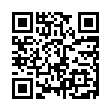# North Coast Synthesis Ltd.

## A+2+4+♯2Choose audio sample set:
MSK 007 Leapfrog VCF (patch; see in online store)
MSK 009 Coiler VCF (patch; see in online store)

Equivalent chord symbols: A+4+9+♯2, A+2+11+♯2.

Notes in this chord: A, B, B♯, C♯, D, E. Integer notation: {0, 1, 2, 4, 9, 11}.

Nearby chords (one less note): A+2+4, A+2+♯2, A+4+♯2, Am+2+4, A4+2+♯2, Bm4+2+♯1.

Nearby chords (one more note): A11+♯2, AM11+♯2, Bm11+♯1, G13-1+♯4, A+2+4+♯1+♯2, A+2+4+♯2+♯4.

Parallel chords (same structure, different root): C+2+4+♯2, D+2+4+♯2, E+2+4+♯2, F+2+4+♯2, G+2+4+♯2, B+2+4+♯2, C♭+2+4+♯2, D♭+2+4+♯2, E♭+2+4+♯2, F♭+2+4+♯2, G♭+2+4+♯2, A♭+2+4+♯2, B♭+2+4+♯2, C♯+2+4+♯2, D♯+2+4+♯2, E♯+2+4+♯2, F♯+2+4+♯2, G♯+2+4+♯2, A♯+2+4+♯2, B♯+2+4+♯2.

Experimental fretting charts for guitar standard EADGBE tuning (change tuning or instrument):